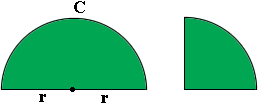SEARCH HOMEMath Central Quandaries & QueriesQuestion from muhammad, a student: a boy has two pieces of wire each 99cm long .he bends them into the shapes shown below. calculate the radius of each figure,giving each answer to two decimal places. figures are one is semicircle and other on is half of the semicircle.Let me start with the semicircle. I see the piece of wire in three parts, two parts are of length $r,$ the length of the radius of the circle, and the third curved part of length $C.$ This curved part is half the circumference of the circle and you know the length of the circumference of the circle is $2 \pi r.$ Thus the total length of the wire is $2 r + 2 \pi r.$ But you know the length of the wire is 99 cm so

$2 r + 2 \pi r = 99$

Solve for $r.$

Now you try the quarter circle.

HarleyMath Central is supported by the University of Regina and The Pacific Institute for the Mathematical Sciences.